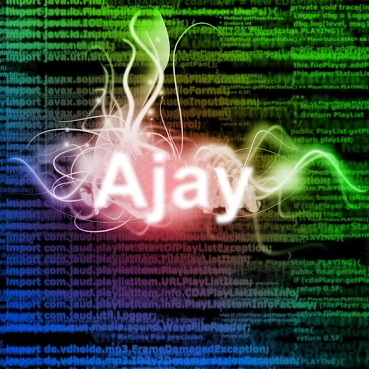Ajay Ramachandran

# Building Hills | Building Roller Coasters | Voster Coaster | VR Game Programming

## July 22, 2018

https://youtu.be/XGmVYe82HlQ

# Repository

https://github.com/ajayyy/VosterCoasterVR

# InclinesInclines are created similarly to turns, except they are rotated upward.

The big issue with this is that the rotation used in all the math had to be changed. Before, only the Y axis was used in math, with inclines, it had to check the x axis.

### Problems with the X axis (Gimbal Lock)

Rotations in game engines are stored as Quaternions, not angles. With euler angles (3d angles), multiple angles can represent the same angle. Ex. (110, 0, 0) and (70, 180, 180). This causes big issues with the math, as 70 degrees is not the proper angle to do math on in this instance. Luckily this is fixable. I luckily found a fix on unity answers, but I still found this phenomenon very confusing and interesting, so I thought I would share my experience here.

https://github.com/ajayyy/VosterCoaster/commit/7fa09d1cf8e192f17a525f80ca27feb628fc30bc

# Creating a full roller coaster

A roller coaster is not just flat with a bunch or turns, or many hills but no turns. A roller coaster has a nice combination of both. To achieve this, the user has to be able to create turns on top of inclines, and inclines after a turn.

### Problems with using one axis for rotation (Making inclines work after a turn)

Using the X axis for rotation works perfectly normally, but completely breaks once an incline needs to be created after a turn, as this moves the axis of rotation of each track piece.

I tried to rotate the angles by adding and subtracting angles, but that turned out not working at all as what needed to happen was axis shifts instead.

After a lot of thinking, I decided to model the situation in a 3D painting program (Tilt Brush), and figured out a solution.I figured out that the axis changes could be discovered using sin and cosine. By looking the rotations and positions of the track for 4 situations (0 degrees, 90, 180, 270), I was able to find the pattern.

``````//adjust angle to make it like it was normal
float angle = Mathf.Cos(currentAngle.y * Mathf.Deg2Rad) * fullTargetAngle.x + Mathf.Sin(currentAngle.y * Mathf.Deg2Rad + Mathf.PI) * fullTargetAngle.z;

targetAngle = new Vector3(angle, 0, 0);``````

The original angle could be found this way. Here is a list of the angles at the 4 angles mentioned above.(top X, bottom Z)

``````45  0  -45  0
0  45  0  45``````

So to find the original angles you can use

``````0  45   90   45
0 -45    0  -45``````

These numbers can be represented by sin and cosine waves.

This same process was used to find the positions at the rotations

``````//theoretical position as if it was at a normal position
float x = Mathf.Sin(currentAngle.y * Mathf.Deg2Rad) * targetPosition.x + Mathf.Cos(currentAngle.y * Mathf.Deg2Rad) * targetPosition.z;
float y = targetPosition.y;

//set that position so that future calculations use that position instead
targetPosition = new Vector3(0, y, x);``````

### Turns after an incline

Luckily, this was much easier to implement. All turns need to do is be at the same height as the previous track, and make sure not to take the previous angle in to effect when creating the track, or else the turn will turn into an incline. Instead, it ignores that angle and only checks for the Y axis angle.

I hope in the future to make it possible to make barrel rolling tracks (turn to the side instead of up or down).

# Automatic Straight Tracks

The only way to make a straight track would be to somehow balance your hand steady enough to form a straight angle. This is practically impossible, so I added a button to force a straight path when needed.

# Auto-complete

I also added basic auto complete functionality. It will automatically set the first track piece as the target position and rotation so that the track will turn toward the original start track and finish it. It will then disable editing mode.

# Pull Request

https://github.com/ajayyy/VosterCoaster/pull/13

You can see the full set of changes in the pull request. This post only includes an overview of major changes.

# GitHub Account

https://github.com/ajayyy